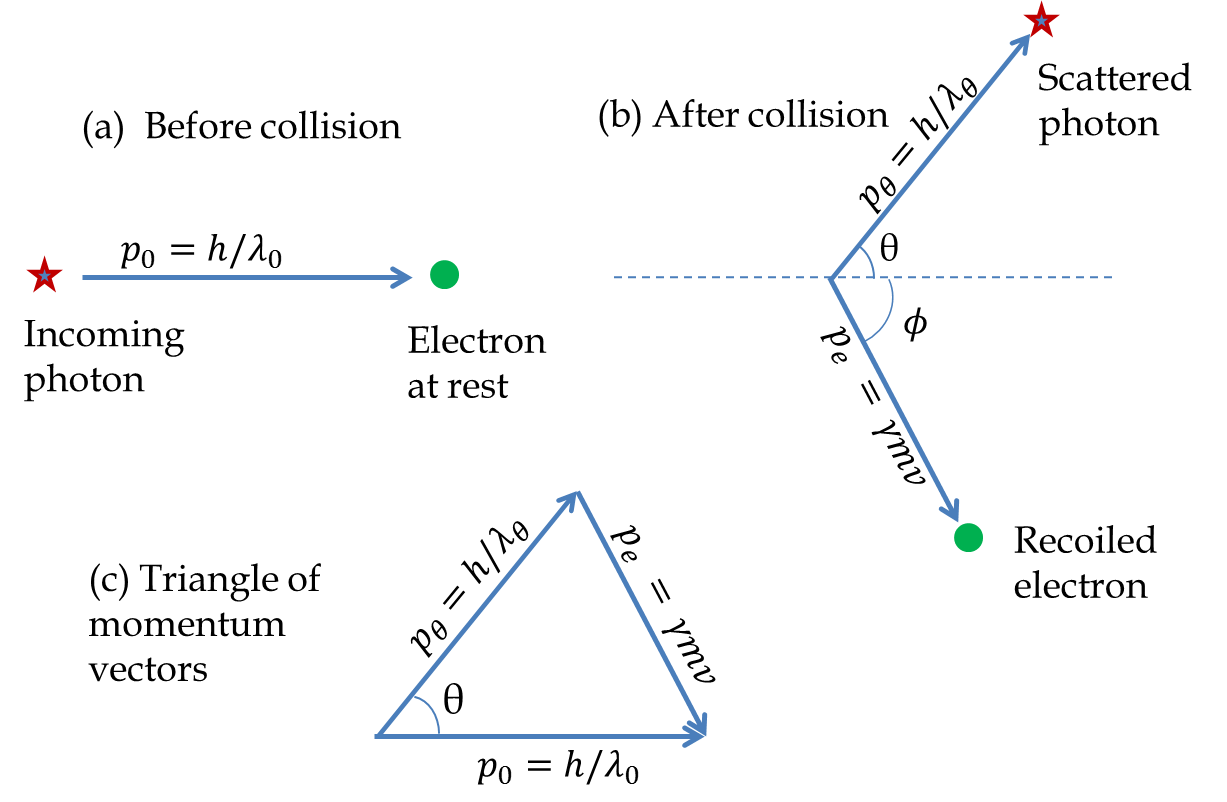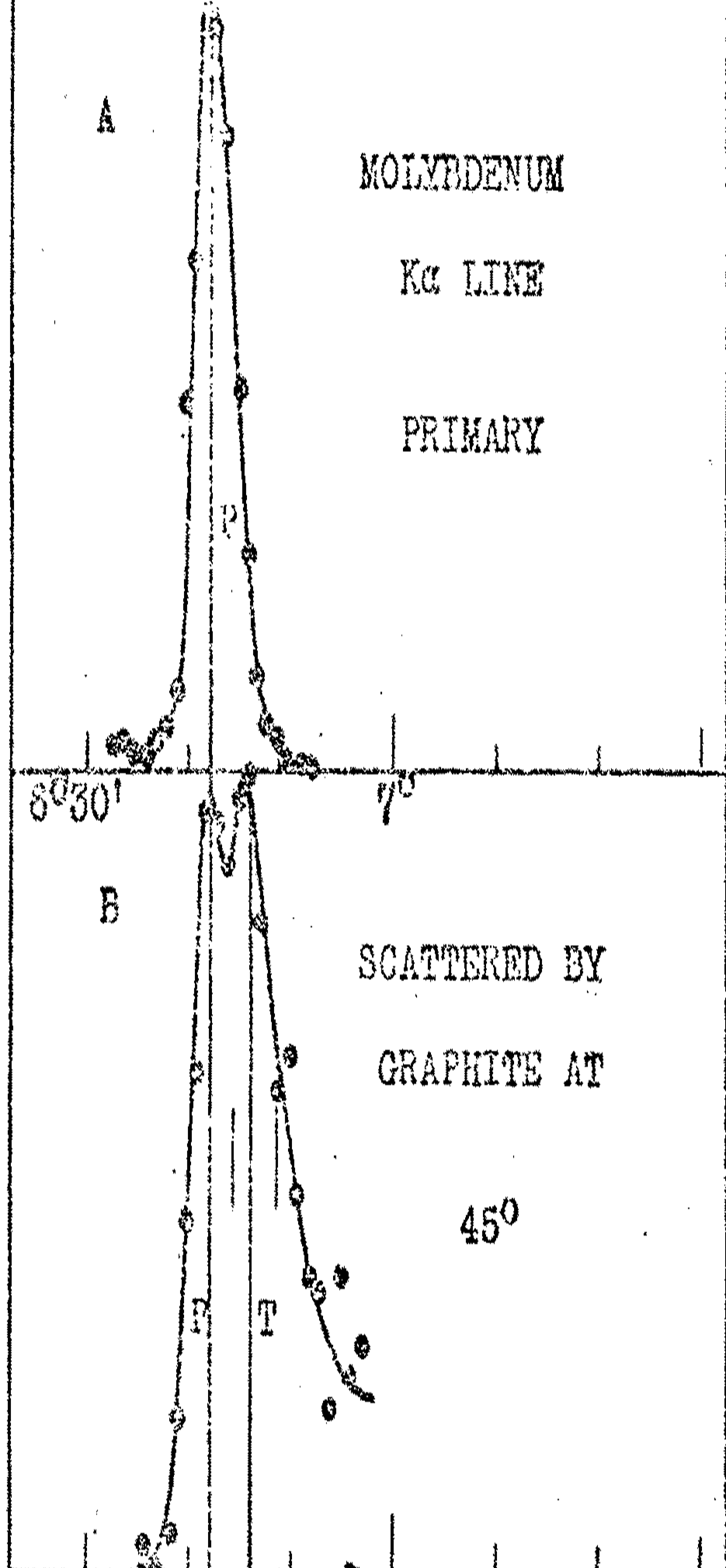## Section53.5Compton Effect

Photoelectric effect demonstrated that energy in light came in bunches or quanta called photons. If photons really do exist as particles, each photon would have energy as well as momentum. We have seen that the energy of a photon is equal to $hf\text{.}$ Since a photon must travel at the speed of light, its rest mass must be zero as shown in the chapter on relativity. This gives the following expression for the momentum of a photon.

\begin{equation} p = \dfrac{E}{c} = \dfrac{h f}{c} = \dfrac{h}{\lambda},\tag{53.5.1} \end{equation}

where I have replaced $f/c = \lambda\text{,}$ where $\lambda$ is the wavelength of the electromagnetic wave. We will often refer to $f$ and $\lambda$ the frequency and wavelength of the photon, even though they refer to the frequency and wavelength of the electromagnetic wave that the photon belongs to.

Arthur Compton of Washington University in St. Louis, Missouri analyzed the energy and momentum conservation in the process of collision of a photon with an electron and found that, the photon coming at an angle to the original direction after collision will have a different wavelength than the wavelength of the incident photon. The predicted shift in wavelength was small, of the order of picometers, and was independent of the wavelength of the incoming photon. Since X-rays have wavelengths in the picometer range the experiment is usually done with X-rays as Compton had done. Also, since the work function of a surface is in the electron-volt (eV) range while the energy of a photon in X-ray has energy in the keV range, we ignore the work function completely.

Collision of a photon with a free electron:

Figure 53.5.1 shows schematically the situation before collision and after collision. The electron initially is taken to be an almost free electron and at rest. This assumption for the electron is justified since the speeds of the photon and the recoiled electron will be in the relativistic regime while the speeds of a loosely bound electron in the material will be non-relativistic.Figure 53.5.1. Collision of a photon with an electron at rest.

Let $\vec p_0$ be the momentum of the incoming photon, $\vec p_\theta$ the momentum of the outgoing photon at angle $\theta\text{,}$ and $\vec p_e$ be the momentum of the recoiled electron. The vector equation of conservation of momentum will be

\begin{equation} \vec p_0 = \vec p_{\theta} + \vec p_e.\tag{53.5.2} \end{equation}

These vectors form a triangle as shown in Figure 53.5.1. The law of cosine applied to the triangle gives the following relation between the magnitudes of the momenta and angle $\theta\text{.}$

\begin{equation} p_e^2 = p_0^2 + p_{\theta}^{2} + 2 p_0 p_{\theta}\cos\theta,\tag{53.5.3} \end{equation}

with the momenta given by the following:

\begin{equation} p_0 = \frac{h}{\lambda_0}\ ,\quad p_{\theta} = \frac{h}{\lambda_{\theta}}\ ,\quad p_e = \gamma m v\ ,\quad \gamma = \frac{1}{\sqrt{1-v^2/c^2}},\tag{53.5.4} \end{equation}

where $v$ is the speed of the recoiled electron, $\lambda_0$ is the wavelength of the incoming photon, and $\lambda_\theta$ the wavelength of the outgoing photon at angle $\theta\text{.}$ Therefore, we get the following equation from the conservation of momentum

\begin{equation} \frac{m^2 v^2}{1-v^2/c^2} = \left(\frac{h}{\lambda_0}\right)^2 + \left(\frac{h}{\lambda_{\theta}}\right)^2 -2 \frac{h^2}{\lambda_0\lambda_{\theta}}\cos\theta. \label{eq-compton-momentum}\tag{53.5.5} \end{equation}

The conservation of energy will give

\begin{equation} \frac{hc}{\lambda_0} + mc^2 = \frac{hc}{\lambda_{\theta}} + \frac{mc^2}{\sqrt{1-v^2/c^2}}.\label{eq-compton-energy}\tag{53.5.6} \end{equation}

Since the velocity of the recoiled electron is not measured in the experiment we will eliminate $v$ from Eqs. (53.5.5) and (53.5.6). A long and tedious calculation gives the following result.

\begin{equation} \lambda_{\theta} - \lambda_0 = \frac{h}{mc} ( 1 - \cos\theta). \label{eq-compton-formula}\tag{53.5.7} \end{equation}

The quantity $\Delta\lambda = \lambda_{\theta} - \lambda_0$ is called the Compton shift and the quantity $\lambda_c = \dfrac{h}{mc}$ is called the Compton wavelength of the particle of mass $m\text{,}$ which is the mass of electron here. Putting the value of $h\text{,}$ $c\text{,}$ and $m$ in $\dfrac{h}{mc}$ we find the Compton wavelength of an electron to be

\begin{align*} \lambda_c \amp = \frac{h}{mc} = \frac{6.63\times 10^{-34}\textrm{J.s}}{9.11\times 10^{-31}\textrm{kg} \times 3.0\times 10^{8}\textrm{m}}\\ \amp = 2.43\times 10^{-12}\:\textrm{m} = 2.43\: \textrm{pm}. \end{align*}

According to Equation (53.5.7) no shift will be seen in the forward direction, $\theta = 0$ and a maximum shift will occur at the back scattered direction, $\theta = 180^{\circ}\text{.}$ At $90^{\circ}$ the shift will equal the Compton wavelength of the object that the photon was scattered from.

### Subsection53.5.1Compton's Experiment

Compton tested Eq. (53.5.7) experimentally using $\text{K}\alpha$ X-rays from a Molybdenum source of wavelength $\lambda = 71.1\:\textrm{pm}\text{.}$ A schematic diagram of the apparatus is shown in Figure 53.5.2. Compton directed the $\text{K}\alpha$ rays to a carbon target. He then observed the scattered rays at various angles with a Bragg spectrometer which consisted of a rotating calcite crystal. The calcite crystal diffracted X-rays of a particular wavelength into an ionization chamber where the intensity of the ray was recorded. From the crystal spacing of the calcite and the angle of diffraction one could determine the wavelength diffracted into the ionization chamber accurately to within $0.1\:\textrm{pm}\text{.}$

Figure 53.5.3 shows Compton's results for scattering at $0^{\circ}, 45^{\circ}, 90^{\circ}\text{,}$ and $135^{\circ}$ angles. The $x$-axis on the figure is the glancing angle from the calcite crystal which is used to calculate the wavelength of X-ray. The experiment at non-zero angles show two peaks, which Compton called the modified and unmodified rays. The peak of the unmodified ray occurs at nearly the same wavelength as the $\text{K}\alpha$ ray from the source while the peak of the modified ray occurs at a longer wavelength in accordance with the formula in Eq. (53.5.7) For instance, the shift at $90^{\circ}$ was found to be $2.2\:\textrm{pm}$ which was in excellent agreement with the predicted shift of $2.4\:\textrm{pm}\text{.}$Figure 53.5.3. The spectrum of $\text{K}\alpha$ rays from Molybdenum at angles $0^{\circ}, 45^{\circ}, 90^{\circ}, 135^{\circ}\text{.}$ The $x$-axis is the glancing angle from the calcite crystal from which the wavelength can be computed. the solid line is the theoretical expectation based on Eq. (53.5.7).

From Eq. (53.5.7) only one peak should appear in any one direction for scattering from one particle. Why do we see two peaks at every angle? Compton investigated this question also. In Eq. (53.5.7) the shift is inversely proportional to the mass $m$ of the particle with which the photon collides. If photon collides with more tightly bound electrons such that the momentum imparted to the electron goes into moving the entire carbon atom, then $m$ in this formula will have to be replaced by mass of the atom, which is approximately 22,000 times heavier than a proton. Therefore, the shift for a collision with a tightly bound electron will be 22,000 times less, meaning if the shift for a loosely bound electron was $2.4\text{ pm}\text{,}$ the shift for tightly bound electron will be $0.11$ fm, which would be not observable in the experiment.

The experimental verification of the Compton effect in 1923 provided strong evidence for the particle nature of light in addition to the photoelectric effect. This helped convince most physicists of the time that the interaction of light with matter cannot be explained by purely appealing to the wave nature of light and that light consisted of stream of particles whose energy was proportional to the frequency of light. For his discovery Compton was awarded the 1927 Nobel prize in physics.

The $\text{K}\alpha_1$ X-ray of wavelength $56.0$ pm from Silver is incident on a graphite target and the scattered ray at $60^{\circ}$ is observed. (a) What will be the Compton shift? (b) What will be the wavelength of the X-ray detected at $60^{\circ}\text{?}$

Hint

(a) Use wavelength shift formula.

(a) $1.215\:\textrm{pm}\text{,}$ (b) $57.215\:\textrm{pm}\text{.}$

Solution

(a) The Compton shift is independent of the wavelength of the incident ray. We can calculate by using the shift formula directly.

\begin{equation*} \Delta \lambda = \lambda_c(1- \cos\theta) = 2.43\:\textrm{pm} (1 - \cos 60^{\circ}) = 1.215\:\textrm{pm}. \end{equation*}

(b) The wavelength observed is $\lambda_{\theta}\text{.}$

\begin{equation*} \lambda_{\theta} = \lambda_0 + \Delta \lambda = 56.0\:\textrm{pm} + 1.215\:\textrm{pm} = 57.215\:\textrm{pm}. \end{equation*}

X-rays of wavelength 21 \text{ pm} from tungsten are incident on a graphite target, which can be considered to contain free electrons. (a) Find the Compton shift at angles (i) $30^{\circ}\text{,}$ (ii) $60^{\circ}\text{,}$ (iii) $90^{\circ}\text{,}$ and (iv) $120^{\circ}\text{.}$ (b) Find the wavelength of the X-rays at angles (i) $30^{\circ}\text{,}$ (ii) $60^{\circ}\text{,}$ (iii) $90^{\circ}\text{,}$ and (iv) $120^{\circ}\text{.}$

X-rays of wavelength $71 \text{ pm}$ from molybdenum are incident on a graphite target and observations are made at $90^{\circ}$ to the original direction. It is found that the detected photon has less energy than the incident X-ray due to Compton scattering. What are the (a) energy and (b) momentum of the scattered electron assuming the scattered electron to be at rest and zero binding energy.

Light of various wavelengths are incident on a free electron target and the scattered light is observed at $60^{\circ}$ to the original direction. (a) What will be the wavelengths of the scattered light if the incident light has the following wavelengths: (i) $2 \text{ cm}$ (Microwave), (ii) $550 \text{ nm}$ (visible), (iii) $10 \text{ nm}$ (uv), (iv) $21 \text{ pm}$ (X-ray), (v) $100 \text{ fm}$ (Gamma ray). (b) What is the percentage change in energy of the photon in each case? (c) What is the percentage change in momentum of the photon in each case?

A $100\text{ keV}$ X-ray scatters off a free electron target and the scattered light is observed at $90^{\circ}$ to the original direction. (a) What will be the wavelength of the scattered light. (b) What is the percentage change in energy of the photon? (c) What is the percentage change in momentum of the photon?

A $100 \text{ GeV}$ gamma-ray scatters off a free electron target and the scattered light is observed at $90^{\circ}$ to the original direction. (a) What will be the wavelength of the scattered light. (b) What is the percentage change in energy of the photon? (c) What is the percentage change in momentum of the photon?